#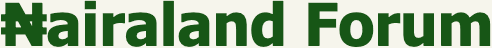Welcome, Guest: Join Nairaland / LOGIN! / Trending / Recent / New
Stats: 2,622,579 members, 6,113,055 topics. Date: Friday, 22 January 2021 at 01:41 PM

## Why Is Any Number Multiplied By Zero Equals To Zero? - Education (3) - Nairaland

 Re: Why Is Any Number Multiplied By Zero Equals To Zero? by ofiko123(m): 12:28pm On Jan 14 JAMB QUESTION.. Re: Why Is Any Number Multiplied By Zero Equals To Zero? by Quoran: 12:28pm On Jan 14 Always write the source of your answers. You copied this from Quora. STINOS:First the commonsense explanation:Say you are giving away free apples. An infinitely big apple tree has sprouted in your garden (it's hypothetical) and you have decided to give away apples to each person who comes by. You have decided that you'll give 1 apple to each person that comes by. If one person comes by, you have given away one apple. If two people come by, you have given away two apples. If seventy-five people come by you have given away seventy-five apples. This is multiplication by 1. If there are n people that come by, 1*n tells you how many apples you have given away.Say you are giving away 2 apples per person. If one person comes by, you have given away two apples. If two people come by you have given away four apples. If thirty people come by you have given away sixty apples. This is multiplication by 2. So if 'n' is how many people came by, 2*n tells you how many apples you have given away.Similarly, if you are giving away 100 apples per person, 100*n tells you how many apples you have given away in total where 'n' is how many people came by to collect their apples (everyone loves apples).If you are giving away 0 apples per person, then regardless of how many people come by, how many apples would you have given out by the end of the day? Logically? None, of course! Hence, 0*n = 0.This also works the other way around, because of the commutative law for multiplication. So lets say no people come by, i.e., n = 0. Regardless of how many apples you want to give away, the total number of apples you have given away will always be 0. Cause no one comes by to take the apples! So a*0 = 0 where a is how many apples you give away per person, which doesn't matter.Now for an explanation hinging slightly more on mathematical notation:Multiplication is basically addition, done over and over again. Multiplying a number n by 2 means adding n twice --> n + nMultiplying a number by 7 means adding it seven times --> n + n + n + n + n + n + nMultiplying a number a gazillion times means n + n + n ... (a gazillion n's)So what you multiply 'n' by tells you the number of n you have to add together. If you multiply n by 0, then there are no n's in the picture. Since you are not adding anything, you are left with nothing, aka 0. So:2*n = n + n (two n's on this side)7*n = n + n + n + n + n + n + n (seven n's on this side)0*n = (how many n's do you see on this side? None. 3 Likes Re: Why Is Any Number Multiplied By Zero Equals To Zero? by Brunicekid(m): 12:28pm On Jan 14 sojfarm:Because anything associated with zero must become zeroJust like you can give what you don't haveI disagree... In Mathematics, the only exception to this rule is index. Any number raised to the power of zero (index of zero) equals one. Re: Why Is Any Number Multiplied By Zero Equals To Zero? by SimplyFacts: 12:28pm On Jan 14 eastOFwest:Lol! You made me laugh. Look at it this way. If you have no money (Zero Naira) and all your brothers, sisters, relatives, extended relatives etc all have no money (Zero Naira), even if there is a hundred of you all together, the combination of all your money is still no money (Zero Naira). Mathematically put - 0 x100 = 0.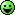Confirmed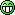Re: Why Is Any Number Multiplied By Zero Equals To Zero? by espn(m): 12:29pm On Jan 14 BobFischer:Why is any any number multiply by zero is equal to zero? 10 x 0 = 0100 x 0 = 01000 x 0 = 0If i have 100 oranges on a table and i multiply it by zero. Why do i suddenly have zero?100 oranges X 0 = 01000 pears X 0 = 0front page pleasemathematicians in the house please gather.2 x 10 =2010 x 2=20Did anything change? 1 Like 1 Share Re: Why Is Any Number Multiplied By Zero Equals To Zero? by Omezif(m): 12:29pm On Jan 14 Shouldn't worry nothing can change it. Re: Why Is Any Number Multiplied By Zero Equals To Zero? by kponkedenge(m): 12:30pm On Jan 14 CalliDora1:My own reasoning is thisWe all know 0 is nothing. Now if you put nothing in ten places, youll get nothing in those ten places. Whic equals to =0/ nothing. So here 10 is represented by place.Hope I've not ended up confusing you further.My head is already paining me reading this chat... And you compounded it.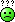Re: Why Is Any Number Multiplied By Zero Equals To Zero? by dexoubie(m): 12:31pm On Jan 14 sinkhole:add no orange (0) ten times (10x), that is 10x0, what will you get?ORadd ten oranges at no time, what will you get?(Note that multiplication is simply repeated addition)Flesh and blood did not reveal this to you bruhRe: Why Is Any Number Multiplied By Zero Equals To Zero? by Martinez39s(m): 12:31pm On Jan 14 0 × 3 means zero is three places. Counting zero in three places gives you zero. Since multiplication is cumulative, anything times zero will give you zero. Re: Why Is Any Number Multiplied By Zero Equals To Zero? by fuckaholic25(f): 12:31pm On Jan 14 I don't care coz since inception, it doesn't help my acct balance!!! Re: Why Is Any Number Multiplied By Zero Equals To Zero? by stealmatic(m): 12:31pm On Jan 14 BobFischer: mind you 0 * 3 and 3 * 0 are two different statement. 0 (nothing) * 3 (something) = 0 (nothing) 3 (something) * 0 (nothing) = 0 (nothing). why isnt it 3 * 0 = 3 0 * 3 = 0 i got ur point am learning Re: Why Is Any Number Multiplied By Zero Equals To Zero? by InvertedHammer: 12:31pm On Jan 14 BobFischer:Why is any any number multiply by zero is equal to zero? 10 x 0 = 0100 x 0 = 01000 x 0 = 0If i have 100 oranges on a table and i multiply it by zero. Why do i suddenly have zero?100 oranges X 0 = 01000 pears X 0 = 0front page pleasemathematicians in the house please gather./If you are to receive N100k many times but you received nothing (0). What is the total amount received? Zero! No matter the number, e no dey means e no dey./ 1 Like Re: Why Is Any Number Multiplied By Zero Equals To Zero? by CalliDora1(f): 12:32pm On Jan 14 kponkedenge:My head is already paining me reading this chat... And you compounded it.LolRe: Why Is Any Number Multiplied By Zero Equals To Zero? by kponkedenge(m): 12:32pm On Jan 14 eastOFwest:Lol! You made me laugh. Look at it this way. If you have no money (Zero Naira) and all your brothers, sisters, relatives, extended relatives etc all have no money (Zero Naira), even if there is a hundred of you all together, the combination of all your money is still no money (Zero Naira). Mathematically put - 0 x100 = 0.This is the most basic explanation so far on this thread for a dullard. 1 Like Re: Why Is Any Number Multiplied By Zero Equals To Zero? by zieraw2005(m): 12:32pm On Jan 14 Hmmmm na so the law be Re: Why Is Any Number Multiplied By Zero Equals To Zero? by UDUJ(m): 12:33pm On Jan 14 Zero means nothing. That means it's not in existence.100 times nothingness or a million times nothingness is still zero.No offense- bros you do arithmetic for primary school soRe: Why Is Any Number Multiplied By Zero Equals To Zero? by jpawjpaw(m): 12:34pm On Jan 14 everglaze01:If somebody send u an alert of 0 naira 100 times. How much do u now have?This life no balanceRe: Why Is Any Number Multiplied By Zero Equals To Zero? by Itzurboi(m): 12:34pm On Jan 14 cos "0" nah chopper, e dey chop anything wey come hin way....Black hole theory comes to mind, 0 on a multiplication level is capable of swallowing the greater number and pretend nothing passed through it...Re: Why Is Any Number Multiplied By Zero Equals To Zero? by S1MP: 12:34pm On Jan 14 Bioles1187:Please delete d screenshot...cc S1MPDone sir Re: Why Is Any Number Multiplied By Zero Equals To Zero? by nairaman66(m): 12:34pm On Jan 14 BobFischer:This translate to zero 0 (if i dont have 1 naira) * 1 or * 100 = 0 (i still dont have any)NOW THIS(You have) 100 * 0 (nothing) = 0 (nothing)translate to you have 100 and i ask zero time(simply i dont ask) and then you loose your 100so how do you explain this?losing you 100 when you do nothingAn example of 'mathematical proof'I realize you don't want too much mathematics, but I'd like to point out that it is rather trivial to "prove", using only the basic few axioms (up to the distributive law)I suppose you do not need much convincing that 0 + 0 = 0 (which itself is a consequence of the additive identity n + 0 = a, with a = 0)Now, as per the distributive law, n * (b+c) = n*b + n*c(b and c are two other arbitrary numbers. So there are b oranges and c apples in a bag. A bag is (b+c). If you buy 2 bags, then you get twice the number of oranges and twice the number of apples --> 2(b+c) = 2b + 2c.)And so n*0 = n* (0+0) = n*0 + n*0, (from the previous bit)So n*0 = n*0 + n*0Subtract (n*0) from both sides, giving0 = n*0N.B Quora 1 Like Re: Why Is Any Number Multiplied By Zero Equals To Zero? by toobusy(m): 12:35pm On Jan 14 Worldliness:Zero practically means nothing.So nothing 1 time, nothing 2 times and nothing 1000 times 0r 1million times is still equal to nothing.Eg) if i dont have 1 naira, if you ask me for 1 naira for 1 time or even 100 times, i still dont have any. that is it.. Still doesn't make much sense to me sir/ma........I don't have any problem believing that 0*30=0 because logically one can say that nothing in 30 places will always be nothing,but I find it almost impossible to believe that 30*0 =0......what is the logical explanation to convince a man who has 30# that because his 30# was multiple by nothing (0) and as such he has no money again?.it doesn't add up. 1 Like Re: Why Is Any Number Multiplied By Zero Equals To Zero? by lieNEWS: 12:36pm On Jan 14 BobFischer:mind you 0 * 3 and 3 * 0 are two different statement. 0 (nothing) * 3 (something) = 0 (nothing)3 (something) * 0 (nothing) = 0 (nothing).why isnt it 3 * 0 = 30 * 3 = 0 because in mathematics addition and multiplication are commutative. That is. 1+2 =2+1. Also 1*3=3*1. Re: Why Is Any Number Multiplied By Zero Equals To Zero? by cutepaul(m): 12:36pm On Jan 14 proxitaly:Buhari (something) x Buhari brain (empty or nothing) = nothingThat is why even 1000 tenures of Buhari will leave Naija empty or even worse off. You grab?mad o Re: Why Is Any Number Multiplied By Zero Equals To Zero? by pickatyouu: 12:36pm On Jan 14 It's simple maths Can u put something into nothing Re: Why Is Any Number Multiplied By Zero Equals To Zero? by Itzurboi(m): 12:37pm On Jan 14 Fortune109:It's just simple English or any other languageThey said...If you don't have 100, what do you have??If you don't have 1000, what do you have??Answer those questions and get your answer!If you don't have 100 naira, then you have like 93naira cos of bank deduction...same applies to 1000naira...Hope you grab my dear bro... Re: Why Is Any Number Multiplied By Zero Equals To Zero? by UDUJ(m): 12:37pm On Jan 14 nairaman66:An example of 'mathematical proof'I realize you don't want too much mathematics, but I'd like to point out that it is rather trivial to "prove", using only the basic few axioms (up to the distributive law)I suppose you do not need much convincing that 0 + 0 = 0 (which itself is a consequence of the additive identity n + 0 = a, with a = 0)Now, as per the distributive law, n * (b+c) = n*b + n*c(b and c are two other arbitrary numbers. So there are b oranges and c apples in a bag. A bag is (b+c). If you buy 2 bags, then you get twice the number of oranges and twice the number of apples --> 2(b+c) = 2b + 2c.)And so n*0 = n* (0+0) = n*0 + n*0, (from the previous bit)So n*0 = n*0 + n*0Subtract (n*0) from both sides, giving0 = n*0N.B Quora Respect for quoting your source QUORA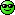Others will steal ideas from the Internet and claim it as theirs. Respect broRe: Why Is Any Number Multiplied By Zero Equals To Zero? by Fiscus105: 12:39pm On Jan 14 BobFischer:mind you 0 * 3 and 3 * 0 are two different statement. 0 (nothing) * 3 (something) = 0 (nothing)3 (something) * 0 (nothing) = 0 (nothing).why isnt it 3 * 0 = 30 * 3 = 0Thank God u illustrated by nothing & something. Can you get something from nothing? Re: Why Is Any Number Multiplied By Zero Equals To Zero? by phemocheee(m): 12:41pm On Jan 14 OP, I love how you think.You concluded that 0x3 and 3x0 are two different statements and I will like to start my explanation from there.Imagine a friend of yours decided to send you 0naira at 3 different times, how much will hit your account? Definitely 0naira right? Which means you never received any money.Now imagine your friend who has 3 naira decided to send it to you at 0time I.e your friend didn't send the money, so how much will you have? Still 0 naira because the money never actually left your friend's account.So 0×3 or 3x0 will always give you 0Hope this helps. 6 Likes Re: Why Is Any Number Multiplied By Zero Equals To Zero? by emae009(m): 12:41pm On Jan 14 mu2sa2: I don't think op's puzzle has been cracked. 0 × 3 = 0 ( the answer is logical: nothing 3 times is still nothing). But why is3 × 0 =0 (this is illogical: e.g if I have 3 apples and try to multiply it 0 times, I can't, but my 3 apples should remain 3, not 0. In case the puzzle can't be cracked in Queen's English, I beg let somebody try good old pidgin - maybe this will help, because with pidgin you are not constrained by rules of grammar ( just say it any how- the language is immaterial as long as the meaning is plain). Using your apples analogy. Let's assume you have a bag full of apples.Scenario 1: (0 * 3 = 0)If I bring 3 boxes and ask you to "not" put any apple in the boxes. How many apples will be in the boxes? zero right?scenario 2: (1 * 3 = 3)If I bring 3 boxes again and I ask you to put "an" apple in each box. The total number of apples in the boxes will be 3 right? Scenario 3: (2 * 3 = 6)3 boxes and you're ask to put 2 apples in each boxes. The summation of apples in the boxes will be 6 right. Scenario 4: (3 * 0 = 0) If I dont bring any box, but I ask you to put 3 apples each box. How many apples will I have in the box?? None because I didn't provide you with any box. Re: Why Is Any Number Multiplied By Zero Equals To Zero? by UDUJ(m): 12:42pm On Jan 14 toobusy:. Still doesn't make much sense to me sir/ma........I don't have any problem believing that 0*30=0 because logically one can say that nothing in 30 places will always be nothing,but I find it almost impossible to believe that 30*0 =0......what is the logical explanation to convince a man who has 30# that because his 30# was multiple by nothing (0) and as such he has no money again?.it doesn't add up.He never had 30 naira in the first place. In this situation we are imagining multiplying 0 naira in 30 places which gives you zero. Nada. Zero balance, bounced checkI don't know if this makes sense to you shaRe: Why Is Any Number Multiplied By Zero Equals To Zero? by micodon(m): 12:44pm On Jan 14 BobFischer:Why is any any number multiply by zero is equal to zero? 10 x 0 = 0100 x 0 = 01000 x 0 = 0If i have 100 oranges on a table and i multiply it by zero. Why do i suddenly have zero?100 oranges X 0 = 01000 pears X 0 = 0front page pleasemathematicians in the house please gather.If you bring 100 oranges from abuja to lagos zero times, how many oranges did you bring to lagos from abuja? Re: Why Is Any Number Multiplied By Zero Equals To Zero? by Aguilar(m): 12:44pm On Jan 14 Reason is that any number in zero places means nothing which makes it zero.

Viewing this topic: felixalbert(m)

(Go Up)

 Sections: politics (1) business autos (1) jobs (1) career education (1) romance computers phones travel sports fashion health religion celebs tv-movies music-radio literature webmasters programming techmarket Nairaland - Copyright © 2005 - 2021 Oluwaseun Osewa. All rights reserved. See How To Advertise. 137Disclaimer: Every Nairaland member is solely responsible for anything that he/she posts or uploads on Nairaland.